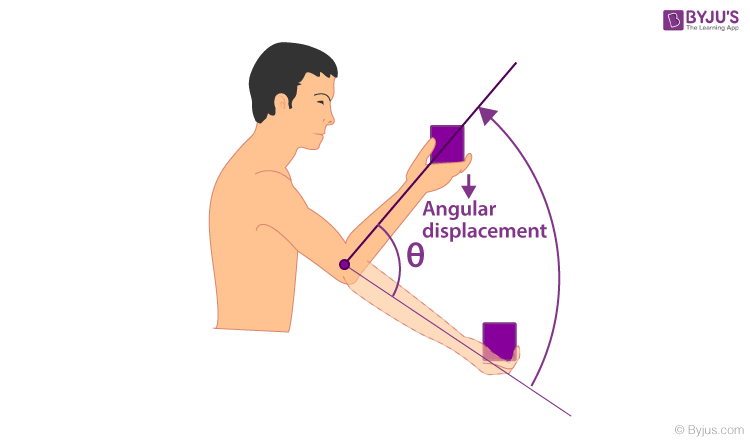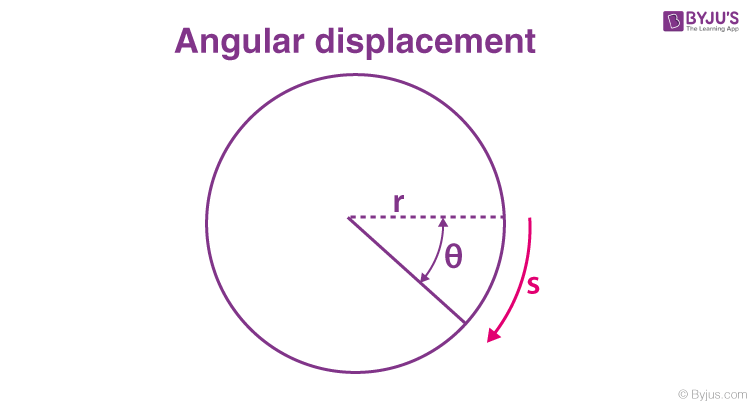Checkout JEE MAINS 2022 Question Paper Analysis : Checkout JEE MAINS 2022 Question Paper Analysis :

# Angular Displacement

In physics, Curvilinear motion is an unavoidable part. The motion of an object along a curved path is studied under circular motion or curvilinear motion. In the case of linear motion, the difference between the initial point and final point is termed as displacement. Thus the circular motion equivalence of displacement is Angular displacement and is represented using Greek letter θ. Angular displacement is measured using the unit degree or radian. In this article, let us know in detail about angular displacement.

## Angular Displacement Definition

Angular displacement is defined as “the angle in radians (degrees, revolutions) through which a point or line has been rotated in a specified sense about a specified axis”. It is the angle of the movement of a body in a circular path.

## What Is Angular Displacement?

It is the angle made by a body while moving in a circular path. Before we go any further into the topic, we have to understand what is meant by rotational motion. When a rigid body is rotating about its own axis, the motion ceases to become a particle. It is so because in a circular path velocity and acceleration can change at any time. The rotation of rigid bodies or bodies which will remain constant throughout the duration of rotation, over a fixed axis is called rotational motion.

The angle made by the body from its point of rest at any point in the rotational motion is the angular displacement.For example- If a dancer dancing around a pole does one full rotation, his or her angular rotation will be

$$\begin{array}{l}360^{o}\end{array}$$
. On the other hand, he or she makes half a rotation; the displacement will be
$$\begin{array}{l}180^{o}\end{array}$$
.

It is a vector quantity, which means that it has both magnitude and direction.

For example, a displacement of

$$\begin{array}{l}360^{o}\end{array}$$
, clockwise is very different from
$$\begin{array}{l}360^{o}\end{array}$$
, counter-clockwise.

### Unit Of Angular Displacement

The unit for this is Radian or Degrees. Two pi radian equals to

$$\begin{array}{l}360^{o}\end{array}$$
. The SI unit of displacement is meter. The angular displacement involves curvilinear motion. SI units of angular displacement is Radian or Degrees.

Measurement of Angular DisplacementIt can be measured by using a simple formula. The formula is:

$$\begin{array}{l}\theta =\frac{s}{r}\end{array}$$
, where,

$$\begin{array}{l}\theta\end{array}$$
is the angular displacement,

s is the distance travelled by the body, and

r is the radius of the circle along which it is moving.

In simpler words, the displacement of an object is the distance travelled by it around the circumference of a circle divided by its radius.

## Frequently Asked Questions – FAQs

### What is angular displacement?

It is the angle in radians through which a point or line has been rotated in a specified sense about a specified axis.

### What is the unit of angular displacement?

Unit of angular displacement is Radian or Degrees.

### Define rotational motion?

The rotation of bodies which will remain constant throughout the duration of rotation, over a fixed axis is known as rotational motion.

### Is angular displacement a scalar or vector quantity?

Angular displacement is a vector quantity since it has both magnitude and direction.

### State true or false: angle made by a body while moving in a circular path is known as angular displacement.

True.

Stay tuned with BYJU’S for more such interesting articles. Also, register to “BYJU’S – The Learning App” for loads of interactive, engaging Physics-related videos and an unlimited academic assist.

Test your knowledge on Angular Displacement